### Toshinori Okuyama and Benjamin M. Bolker. 2005. Combining genetic and ecological data to estimate esa turtle origins. Ecological Applications 15:315–325.

Appendix A. The model specification and Markov Chain Monte Carlo.

The DAG description of the model is shown in Fig. A1. The covariate model is extended from the previous non-covariate model (Pella and Masuda 2001). The extended components of the model are described here (i.e., the gray nodes in Fig. A1).

Let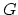(indexed by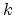) denote the number of gyres. There are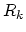rookeries in theth gyre. The size of rookeries in theth gyre is described by vector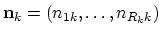.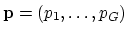is the contribution vector for gyres (i.e., the rookery contributions ofth gyre sum to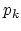). Let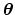be the mixed stock contributions such that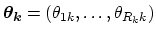is the contribution vector forth gyre;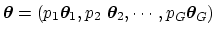where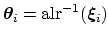. Which follows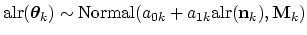where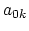and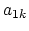are the intercept and slope parameters for theth gyre.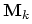is a variance covariance matrix for theth gyre. When performing the additive log ratio (alr) transform, the smallest rookery was taken as the base: i.e., the rookeries were sorted in decreasing order of size, so that the denominator in equation 2 was the smallest rookery size. The prior distribution of the slope parameter was restricted to be nonnegative;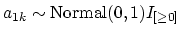. This incorporates our opinion that rookery size and true rookery contribution might be uncorrelated, but would not be negatively correlated. The other priors were set as follows: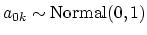,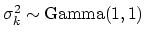where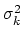are the diagonal elements of the diagonal covariance matrix. These priors have expected values equivalent to the non-covariate model, so that in cases where the data are uninformative about the relationship between rookery size and rookery contribution the model will behave like the non-covariate model. For example, in the simulations presented in this paper, the parameter(slope of the relationship between rookery size and rookery contribution in gyre) had a posterior distribution concentrated around the true slope for the additive-log-ratio transformed sizes and contributions. As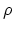, the correlation between the non-transformed sizes and contributions, decreased toward zero,decreased toward zero (equivalent to the non-covariate model) as well. The hyperparameters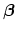(parameters for the distribution of rookery haplotypes) were determined by using the pseudo-Bayes method (Pella and Masuda 2001). We used a Dirichlet prior for the gyre contribution,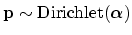where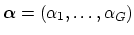. We set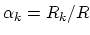which corresponds to distributing equal weight to each rookery, and assuming an amount of prior information equivalent to sampling a single individual (a weak prior).

It is not necessary to incorporate ecological covariates for every gyre. For example, when there are two gyres, a covariate structure can be specified for one gyre and a non-covariate structure can be specified for the other gyre. Such a specification may be useful if the rookery sizes provide useful information in only one gyre. For example, in our analysis with green turtle data, we used the covariate model for both gyres, but for loggerhead turtles, we omitted the covariate model for the second gyre (since it contains only two rookeries). In general we would only specify a covariate model if there were at least four rookeries in a gyre.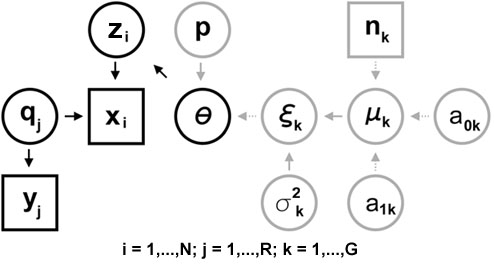FIG. A1. Directed acyclic graph for the stock mixture model. Notation: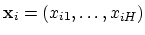is the haplotype profile of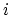th individual in the mixture population such that if the individual's haplotype is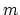,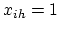for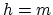and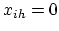for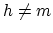.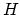denotes the number of observed haplotypes.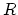denotes the number of rookeries.denotes the number of rookeries in theth gyre (i.e.,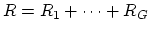).denotes the number of gyres.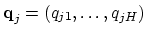is the set of haplotype frequencies for the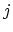th rookery.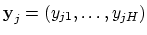is the number of turtles fromth rookery such that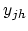denotes the number of turtles with haplotype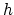in theth rookery.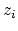indicates the stock identity ofth individual in the mixture population: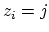indicates theth individual is imputed to theth rookery.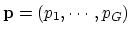is the contributions from each of theocean gyres.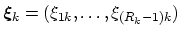is the additive log ratio transformed contribution within theth ocean gyre.is the number of rookeries in theth gyre.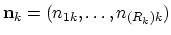is the vector of rookery sizes in theth gyre.

To implement Gibbs sampling, we need to specify the full conditional distribution for each unobserved node. A DAG model provides a way to describe the parameters using the following general relationship: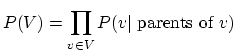where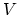is a set of all the node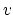, and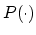is a probability distribution. A full conditional distribution for a node is the distribution of that node given current or known values for all the other nodes in the graph (Spiegelhalter et al. 1996). Let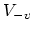be the set of all the nodes except for. Then the full conditional distribution is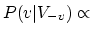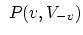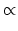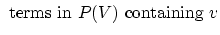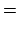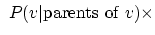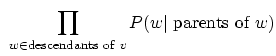where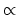means "proportional to". For example, the full conditional distribution for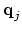is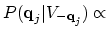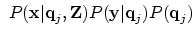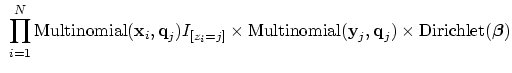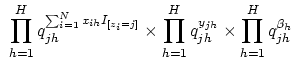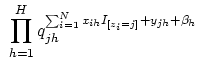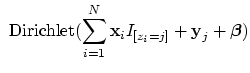where Z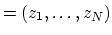and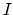is the indicator function.

Once all the full conditional distributions are specified, we can implement a Markov Chain Monte Carlo (MCMC) that will eventually converge on the posterior distributions of all of the parameters and hyperparameters. For a large variety of models (including those described here), this process can be completely automated using a freely available program called BUGS (Bayesian inference Using Gibbs Sampling) (http://www.mrc-bsu.cam.ac.uk/bugs/). MCMC only converges to the appropriate distribution of parameter values in the long run, after the starting values of the parameters have been forgotten". We used standard methods, as implemented in the publicly available CODA package (linked from the BUGS website), to evaluate how long this so-called burn-in" period should be, and how long the chain must be run to get reasonable estimates. For our simulations, we used Gelman-Rubin convergence diagnostics with five overdispersed initial values (Gelman et al. 1996). For all MCMC runs, we took 5000 burn-in and 20000 subsequent runs for the analyses. In the simulations, when the convergence criteria was not met, we discarded the results instead of running for longer for the computational simplicity, but such occasions were very rare and should not bias our results.

LITERATURE CITED

Gelman, A., J. Carlin, H. S. Stern, and D. B. Rubin. 1996. Bayesian data analysis. Chapman and Hall, New York, New York, USA.

Pella, J., and M. Masuda. 2001. Bayesian methods for analysis of stock mixtures from genetic characters. Fisheries Bulletin 99:151–167.

Spiegelhalter, D. J., N. G. Best, W. R. Gilks, and H. Inskip. 1996. Hepatitis B: a case study in MCMC methods. Pages 21–74 in W. R. Gilks, S. Richardson, and D. J. Spiegelhalter, editors. Markov Chain Monte Carlo in practice, Chapman and Hall/CRC, Boca Raton, Florida, USA.

[Back to A015-009]